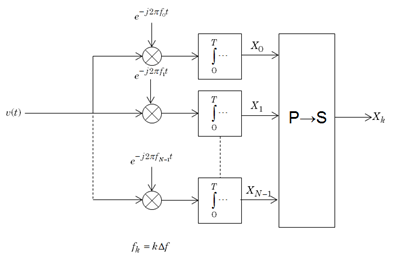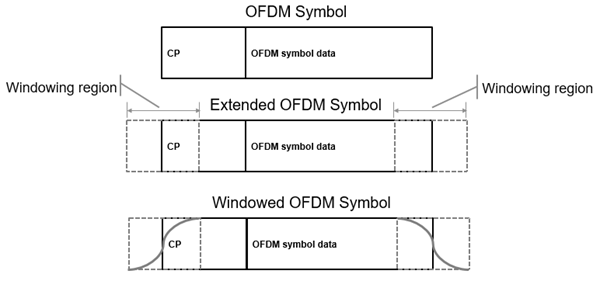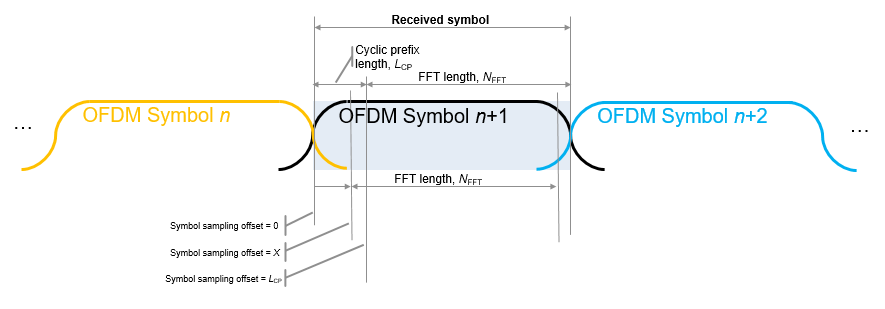Documentation

# ofdmdemod

Demodulate time-domain signal using orthogonal frequency division multiplexing (OFDM)

## Syntax

``outSym = ofdmdemod(ofdmSig,nfft,cplen)``
``outSym = ofdmdemod(ofdmSig,nfft,cplen,symOffset)``
``outSym = ofdmdemod(ofdmSig,nfft,cplen,symOffset,nullidx)``
``[outSym,pilots] = ofdmdemod(ofdmSig,nfft,cplen,symOffset,nullidx,pilotidx)``

## Description

example

````outSym = ofdmdemod(ofdmSig,nfft,cplen)` performs OFDM demodulation on the input time domain signal specified in `ofdmSig`, using an FFT size specified by `nfft` and cyclic prefix length specified by `cplen`. For information, see OFDM Demodulation.```
````outSym = ofdmdemod(ofdmSig,nfft,cplen,symOffset)` applies the symbol sampling offset, `symOffset`, for each OFDM symbol before demodulation of the input.```

example

````outSym = ofdmdemod(ofdmSig,nfft,cplen,symOffset,nullidx)` removes null subcarriers from the locations specified in `nullidx`. For this syntax, the symbol sampling offset is applied to each OFDM symbol and the number of rows in the output is `nfft` – `length(nullidx)`, which accounts for the removal of null subcarriers. Use null subcarriers to account for guard bands and DC subcarriers. For information, see Subcarrier Allocation and Guard Bands.```

example

````[outSym,pilots] = ofdmdemod(ofdmSig,nfft,cplen,symOffset,nullidx,pilotidx)` returns pilot subcarriers for the pilot indices specified in `pilotidx`. For this syntax, the symbol sampling offset is applied to each OFDM symbol and number of rows in the output is `nfft` – `length(nullidx)` – `length(pilotidx)`, which accounts for the removal of null and pilot subcarriers. The function assumes that pilot subcarrier locations are the same across each OFDM symbol and transmit antenna.```

## Examples

collapse all

OFDM-demodulate a signal with different CP lengths for different symbols.

Initialize input parameters defining locations for null and pilot subcarriers. Generate random data and perform OFDM modulation.

```nfft = 64; cplen = [16 32]; nSym = 2; dataIn = complex(randn(nfft,nSym),randn(nfft,nSym)); y1 = ofdmmod(dataIn,nfft,cplen);```

Demodulate the OFDM symbols. Compare the results to the original input data. The difference between the signals is negligible.

```x1 = ofdmdemod(y1,nfft,cplen); max(x1-dataIn)```
```ans = 1×2 complex 10-15 × 0.2220 - 0.7772i 0.2498 - 0.8882i ```

Apply OFDM multiplexing to a 16-QAM signal SISO link with Rayleigh fading.

```s1 = RandStream('mt19937ar','Seed',12345); nFFT = 64; cpLen = 16; nullIdx = [1:6 33 64-4:64].'; numTones = nFFT-length(nullIdx); modOrd = 4; qam = comm.RectangularQAMModulator('ModulationOrder',2^modOrd, ... 'BitInput',true,'NormalizationMethod','Average power'); maxDopp = 1; pathDelays = [0 4e-3 8e-3]; pathGains = [0 -2 -3]; sRate = 1000; sampIdx = round(pathDelays/(1/sRate)) + 1; chan = comm.RayleighChannel('PathGainsOutputPort',true, ... 'MaximumDopplerShift',maxDopp,'PathDelays',pathDelays, ... 'AveragePathGains',pathGains,'SampleRate',sRate, ... 'RandomStream','mt19937ar with seed'); data = randi(s1,[0 1],modOrd*numTones,1); modOut = qam(data); ```

Apply OFDM modulation and pass the signal through the channel.

```y = ofdmmod(modOut,nFFT,cpLen,nullIdx); [fadSig, pg] = chan(y); ```

Determine symbol sampling offset. OFDM demodulate the received signal.

```symOffset = min(max(sampIdx),cpLen) x = ofdmdemod(fadSig,nFFT,cpLen,symOffset,nullIdx); % with a time shift ```
```symOffset = 9 ```

Convert path gains, `pg`, to scalar taps gains. Use the tap gains for equalization during signal recovery.

```hImp = complex(zeros(1,nFFT)); hImp(:,sampIdx) = mean(pg,1); hall = fftshift(fft(hImp.'),1); dataIdx = double(setdiff((1:nFFT)',nullIdx)); h = hall(dataIdx); ```

Equalize the signal.

```eqH = conj(h)./(conj(h).*h); eqSig = eqH.*x; cdScope = comm.ConstellationDiagram('ShowReferenceConstellation',true, ... 'ReferenceConstellation',constellation(qam)); cdScope(eqSig); ```Demodulate the 16-QAM symbols to recover the signal. Compute the bit error rate.

```qamdem = comm.RectangularQAMDemodulator('ModulationOrder',2^modOrd, ... 'BitOutput',true,'NormalizationMethod','Average power'); rxBits = qamdem(eqSig); numErr = biterr(data,rxBits); disp(['Number of bit errors: ' num2str(numErr) ' out of ' num2str(length(data)) ' bits.']) ```
```Number of bit errors: 3 out of 208 bits. ```

OFDM-demodulate data input that includes null and pilot packing.

Initialize input parameters, defining locations for null and pilot subcarriers. Generate random data and perform OFDM modulation.

```nfft = 64; cplen = 16; nSym = 10; nullIdx = [1:6 33 64-4:64]'; pilotIdx = [12 26 40 54]'; numDataCarrs = nfft-length(nullIdx)-length(pilotIdx); dataIn = complex(randn(numDataCarrs,nSym),randn(numDataCarrs,nSym)); pilots = repmat(pskmod((0:3).',4),1,nSym); y2 = ofdmmod(dataIn,nfft,cplen,nullIdx,pilotIdx,pilots); ```

Demodulate the OFDM symbols. Compare the results to the original input data to show that there is negligible difference between the demodulated signal and the original data and pilot signals.

```symOffset = cplen; [x2,rxPilots] = ofdmdemod(y2,nfft,cplen,symOffset,nullIdx,pilotIdx); max(x2-dataIn)```
```ans = 1×10 complex 10-15 × 0.5551 + 0.2220i 0.2220 + 0.4441i 0.4441 - 0.2220i 0.4718 - 0.3331i -0.1665 - 0.4441i -0.3331 - 0.8049i 0.6661 - 0.2220i 0.0000 + 0.5829i 0.2220 + 0.4441i 0.3331 + 0.4441i ```
`max(rxPilots-pilots)`
```ans = 1×10 complex 10-15 × 0.0000 + 0.3331i 0.1837 - 0.2220i -0.4441 - 0.2776i 0.2220 + 0.2220i 0.2220 - 0.1665i 0.0000 - 0.3445i 0.3331 + 0.0441i -0.4441 - 0.1225i 0.4441 + 0.1943i 0.4441 + 0.3192i ```

## Input Arguments

collapse all

Modulated OFDM symbols, specified as a 2-D array of complex symbols.

• If `cplen` is a scalar, the array size is ((`nfft` + `cplen`) × NSym)-by-NR.

• If `cplen` is a row vector, the array size is ((`nfft` × NSym) + `sum`(`cplen`))-by-NR.

NSym is the number of symbols per antenna and NR is the number of receive antennas.

Data Types: `double` | `single`
Complex Number Support: Yes

FFT length, specified as an integer greater than or equal to 8. `nfft` is equivalent to the number of subcarriers used in the demodulation process.

Data Types: `double`

Cyclic prefix length, specified as a scalar or as a row vector of length NSym.

• When you specify `cplen` as a scalar, the cyclic prefix length is the same for all symbols through all antennas.

• When you specify `cplen` as a row vector of length NSym, the cyclic prefix length can vary across symbols but remains the same length through all antennas.

Data Types: `double`

Symbol sampling offset, specified as values from 0 to `cplen`.

• If you do not specify `symOffset`, the default value is an offset equal to `cplen`.

• If you specify `symOffset` as a scalar, the same offset is used for all symbols.

• If you specify `symOffset` as a row vector, the offset value can be different for each symbol.

For information, see Windowing and Symbol Offset.

Data Types: `double`

Indices of null subcarrier locations, specified as a column vector with element values from 1 to `nfft`. If you specify `nullidx`, the number of rows in `outSym` is `(nfft-length(nullidx))`. For information, see Subcarrier Allocation and Guard Bands.

Data Types: `double`

Indices of pilot subcarrier locations, specified as a column vector with element values from 1 to `nfft`. If you specify `pilotidx`, the number of rows in `outSym` is `(nfft-length(nullidx)-length(pilotidx))`.For information, see Subcarrier Allocation and Guard Bands.

Data Types: `double`

## Output Arguments

collapse all

Output demodulated symbols, returned as an ND-by-NSym-by-NR numeric array of symbols. ND must equal `nfft``length(nullidx)``length(pilotidx)`. NSym is the number of OFDM symbols per antenna. NR is the number of receive antennas. For information, see OFDM Demodulation.

Pilot subcarriers, returned as an NPilot-by-NSym-by-NR numeric array of symbols. NPilot must equal the length of `pilotidx`. NSym is the number of OFDM symbols per antenna. NR is the number of receive antennas. The function assumes that the pilot subcarrier locations are the same across each OFDM symbol and transmit antenna. Use the `comm.OFDMDemodulator` to vary pilot subcarrier locations across OFDM symbols or antennas.

collapse all

### OFDM Demodulation

An OFDM demodulator demultiplexes a multi-subcarrier time-domain signal using orthogonal frequency division modulation.

The OFDM demodulation uses an FFT operation that results in N parallel data streams. An OFDM demodulator consists of a bank of N correlators, with one correlator assigned to each OFDM subcarrier, followed by a parallel-to-serial conversion.### Subcarrier Allocation and Guard Bands

Individual OFDM subcarriers are allocated as data, pilot, or null subcarriers.

As shown here, subcarriers are designated as data, DC, pilot, of guard band subcarriers.• Data subcarriers transmit user data.

• Pilot subcarriers are used for channel estimation.

• Null subcarriers transmit no data. Subcarriers with no data are used to provide a DC null and serve as buffers between OFDM resource blocks.

• The null DC subcarrier is the center of the frequency band with an index value of (`nfft`/2 + 1) if `nfft` is even, or ((`nfft` + 1) / 2) if `nfft` is odd.

• The guard bands provide buffers between consecutive OFDM symbols to protect the integrity of transmitted signals by reducing intersymbol interference.

Null subcarriers enable you to model guard bands and DC subcarrier locations for specific standards, such as the various 802.11 formats, LTE, WiMAX, or for custom allocations. You can allocate the location of nulls by assigning a vector of null subcarrier indices.

### Windowing and Symbol Offset

To reduce intersymbol interference (ISI) introduced by signal windowing applied at the transmitter, the function applies a fractional symbol offset before demodulation of each OFDM symbol. Signal windowing is often applied to transmitted OFDM symbols to smooth the discontinuity between consecutive OFDM symbols. Windowing reduces intersymbol out-of-band emissions but increases ISI.

The windowed OFDM symbol consists of the cyclic prefix (CP), ODFM symbol data, plus windowing regions at the beginning and end of the symbol. The leading and trailing windowing shoulders have tails as shown in the figure.To reduce ISI, you can align signal sample timing by specifying a symbol sampling offset that gets applied before OFDM symbol demodulation.Specify the symbol sampling offset as a value from 0 to LCP.

• When the symbol sampling offset is a scalar from 0 to LCP, the FFT window begins at the X+1 sample of the CP length.

• When the symbol sampling offset is zero, no offset is applied and the FFT window starts at the first sample of the symbol.

• When the symbol sampling offset is the cyclic prefix length, LCP, the FFT window begins after the last CP sample. This offset is the default setting if symbol sampling offset is not specified.